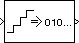Uniform Encoder

Quantize and encode floating-point input into integer output

Library

Quantizers

dspquant2

•Description

The Uniform Encoder block performs the following two operations on each floating-point sample in the input vector or matrix:

1. Quantizes the value using the same precision

2. Encodes the quantized floating-point value to an integer value

In the first step, the block quantizes an input value to one of 2B uniformly spaced levels in the range [-V, (1-21-B)V], where you specify B in the Bits parameter and you specify V in the Peak parameter. The quantization process rounds both positive and negative inputs downward to the nearest quantization level, with the exception of those that fall exactly on a quantization boundary. The real and imaginary components of complex inputs are quantized independently.

The number of bits, B, can be any integer value between 2 and 32, inclusive. Inputs greater than (1-21-B)V or less than -V saturate at those respective values. The real and imaginary components of complex inputs saturate independently.

In the second step, the quantized floating-point value is uniquely mapped (encoded) to one of 2B integer values. When the Output type is set to Unsigned integer, the smallest quantized floating-point value, -V, is mapped to the integer 0, and the largest quantized floating-point value, (1-21-B)V, is mapped to the integer 2B-1. Intermediate quantized floating-point values are linearly (uniformly) mapped to the intermediate integers in the range [0, 2B-1]. For efficiency, the block automatically selects an unsigned output data type (uint8, uint16, or uint32) with the minimum number of bits equal to or greater than B.

When the Output type is set to Signed integer, the smallest quantized floating-point value, -V, is mapped to the integer -2B-1, and the largest quantized floating-point value, (1-21-B)V, is mapped to the integer 2B-1-1. Intermediate quantized floating-point values are linearly mapped to the intermediate integers in the range [-2B-1, 2B-1-1]. The block automatically selects a signed output data type (int8, int16, or int32) with the minimum number of bits equal to or greater than B.

Inputs can be real or complex, double or single precision. The output data types that the block uses are shown in the table below. Note that most of the DSP System Toolbox™ blocks accept only double-precision inputs. Use the Simulink® Data Type Conversion block to convert integer data types to double precision. See About Data Types in Simulink (Simulink) for a complete discussion of data types, as well as a list of Simulink blocks capable of reduced-precision operations.

BitsUnsigned IntegerSigned Integer

2 to 8

uint8

int8

9 to 16

uint16

int16

17 to 32

uint32

int32

The Uniform Encoder block operations adhere to the definition for uniform encoding specified in ITU-T Recommendation G.701.

Examples

See example model ex_uniform_encoder.

In this example, the following parameters are set:

• Peak = 2

• Bits = 3

• Output type = Unsigned

The following figure illustrates uniform encoding.The real and complex components of each input (horizontal axis) are independently quantized to one of 23 distinct levels in the range [-2,1.5]. These components are then mapped to one of 23 integer values in the range [0,7].

-2.0  is mapped to  0
-1.5  is mapped to  1
-1.0  is mapped to  2
-0.5  is mapped to  3
0.0  is mapped to  4
0.5  is mapped to  5
1.0  is mapped to  6
1.5  is mapped to  7

This table shows the results for a few particular inputs.

InputQuantized InputOutputNotes

1.6

1.5+0.0i

7+4i

-0.4

-0.5+0.0i

3+4i

-3.2

-2.0+0.0i

4i

Saturation (real)

0.4-1.2i

0.0-1.5i

4+i

0.4-6.0i

0.0-2.0i

4

Saturation (imaginary)

-4.2+3.5i

-2.0+2.0i

7i

Saturation (real and imaginary)

The output data type is automatically set to uint8, the most efficient format for this input range.

Parameters

Peak

The largest input amplitude to be encoded, V. Real or imaginary input values greater than (1-21-B)V or less than -V saturate (independently for complex inputs) at those limits.

Bits

Specify the number of bits, B, needed to represent the integer output. The number of levels at which the block quantizes the floating-point input is 2B.

Output type

The data type of the block's output, Unsigned integer or Signed integer. Unsigned outputs are uint8, uint16, or uint32, while signed outputs are int8, int16, or int32.

References

General Aspects of Digital Transmission Systems: Vocabulary of Digital Transmission and Multiplexing, and Pulse Code Modulation (PCM) Terms, International Telecommunication Union, ITU-T Recommendation G.701, March, 1993

Supported Data Types

PortSupported Data Types

Input

• Double-precision floating point

• Single-precision floating point

Output

• 8-, 16-, and 32-bit integers

 Data Type Conversion (Simulink) Simulink Quantizer (Simulink) Simulink DSP System Toolbox Uniform Decoder DSP System Toolbox udecode Signal Processing Toolbox uencode Signal Processing Toolbox

Extended Capabilities

C/C++ Code GenerationGenerate C and C++ code using Simulink® Coder™.

Introduced before R2006a

DSP System Toolbox DocumentationGet trial now